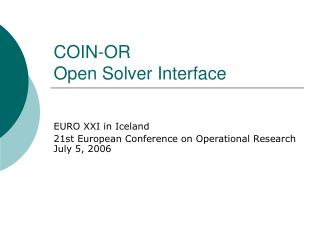Download PresentationCOIN-OR Open Solver Interface

# COIN-OR Open Solver Interface - PowerPoint PPT Presentation

COIN-OR Open Solver Interface. EURO XXI in Iceland 21st European Conference on Operational Research July 5, 2006. Open Solver Interface (OSI). What is the COIN-OR OSI? How is it used? Gotchas Summary. OSI provides a uniform interface to many LP solversI am the owner, or an agent authorized to act on behalf of the owner, of the copyrighted work described.
Download Presentation## COIN-OR Open Solver Interface

An Image/Link below is provided (as is) to download presentation

Download Policy: Content on the Website is provided to you AS IS for your information and personal use and may not be sold / licensed / shared on other websites without getting consent from its author.While downloading, if for some reason you are not able to download a presentation, the publisher may have deleted the file from their server.

- - - - - - - - - - - - - - - - - - - - - - - - - - E N D - - - - - - - - - - - - - - - - - - - - - - - - - -
Presentation Transcript
1. COIN-OROpen Solver Interface EURO XXI in Iceland 21st European Conference on Operational Research July 5, 2006

2. Open Solver Interface (OSI) • What is the COIN-OR OSI? • How is it used? • Gotchas • Summary

3. OSI provides a uniform interface to many LP solvers The goal is to isolate an application from a specific solver. An application written to the OSI should be able to easily change solvers. Clp: OsiClp CPLEX: OsiCpx DyLP: OsiDylp FortMP: OsiFmp GLPK: OsiGlpk MOSEK: OsiMsk OSL: OsiOsl SoPlex: OsiSpx SYMPHONY: OsiSym Vol: OsiVol XPRESS-MP OsiXpr What?

4. How is it used • OSI is a C++ abstract base class • An implementation for a specific solver inherits from the OSI base class. • For example: OsiClp inherits from Osi.

5. Example: Simple LP minimize -1x0 - 1x1 such that 1x0 + 2x1 <= 3 2x0 + 1x1 <= 3 x0 >= 0 x1 >= 0

6. Defining LP Model – obj. & col. bounds //Define objective coefficients. minimize -1 x0 - 1 x1 int n_cols = 2; double *objective = new double[n_cols]; objective = -1.0; objective = -1.0; // Define column bounds // 0 <= x0 <= infinity // 0 <= x1 <= infinity double *col_lb = new double[n_cols]; //the column lower bounds double *col_ub = new double[n_cols]; //the column upper bounds col_lb = 0.0; col_lb = 0.0; OsiSolverInterface *si = new OsiClpSolverInterface; col_ub = si->getInfinity(); col_ub = si->getInfinity();

7. Defining LP Model – rows //Define the constraint matrix. CoinPackedMatrix *matrix = new CoinPackedMatrix(false,0,0); matrix->setDimensions(0, n_cols); // -infinity <= 1 x0 + 2 x2 <= 3 CoinPackedVector row1; row1.insert(0, 1.0); row1.insert(1, 2.0); matrix->appendRow(row1); //-infinity <= 2 x0 + 1 x1 <= 3 CoinPackedVector row2; row2.insert(0, 2.0); row2.insert(1, 1.0); matrix->appendRow(row2); // Define Row Bounds int n_rows = 2; double *row_lb = new double[n_rows];//the row lower bounds double *row_ub = new double[n_rows];//the row upper bounds row_lb = -1.0 * si->getInfinity(); row_ub = 3.0; row_lb = -1.0 * si->getInfinity(); row_ub = 3.0;

8. Pass Model to OSI, Solve, get solution //load the problem to OSI si->loadProblem(*matrix, col_lb, col_ub, objective, row_lb, row_ub); //write the MPS file to a file called example.mps si->writeMps("example"); // Solve the (relaxation of the) problem si->initialSolve(); // Check the solution if ( si->isProvenOptimal() ) { cout <<"Objective value is " <<si->getObjValue() <<endl; int nc = si->getNumCols(); const double *solution = si->getColSolution(); for( int c=0; c<nc; ++c ) { cout <<"x[" <<c <<"]=" <<solution[c] <<endl; } }

9. Example: Cut Generation Library • Read mps file to define model • Solve lp • Generate cuts • If no new cuts generated then done • Add new cuts to model • Resolve LP • If objective function value changes continue with step 3

10. Read MPS file and initial solve OsiClpSolverInterface si; si.readMps(p0033.mps,"mps"); // Solve continuous problem si.initialSolve(); // Instantiate cut generators CglKnapsackCover knapsackCoverCg; CglSimpleRounding simpleRoundingCg;

11. Read MPS file and initial solve do { // Generate and apply cuts OsiCuts cuts; knapsackCoverCg.generateCuts(si,cuts); simpleRoundingCg.generateCuts(si,cuts); OsiSolverInterface::ApplyCutsReturnCode acRc = si.applyCuts(cuts,0.0); // If no cuts were applied, then done if ( acRc.getNumApplied()==0 ) break; // Resolve double obj = si.getObjValue(); // Grab obj value before resolve si.resolve(); cout <<"After applying cuts, objective value changed from " <<obj <<" to " <<si.getObjValue() <<endl; CoinRelFltEq eq(0.0001); bool equalObj = eq( si.getObjValue(), obj ); } while( !equalObj );

12. The Reality • Osi functionality is limited compared to most LP solvers • Depending on the solver and how well the solver’s derived Osi class is written there can be performance overhead • Not all Osi’s behave the same (need better testing and definitions). • Osi provides mechanism to get to the underlying solver

13. More info: www.coin-or.org • These charts: • www.coin-or.org/Presentations/EURO06/ • matrix, vector, floatEqual classes: • projects.coin-or.org/CoinUtils • Osi methods: • projects.coin-or.org/Osi • Cgl classes: • projects.coin-or.org/Cgl • Examples: • projects.coin-or.org/Osi/browser/trunk/Osi/examples • projects.coin-or.org/Cgl/browser/trunk/Cgl/examples